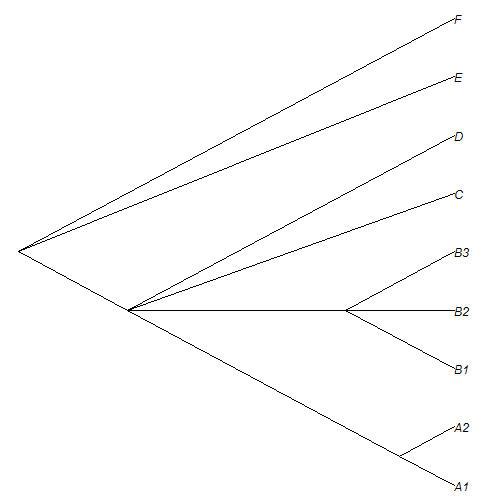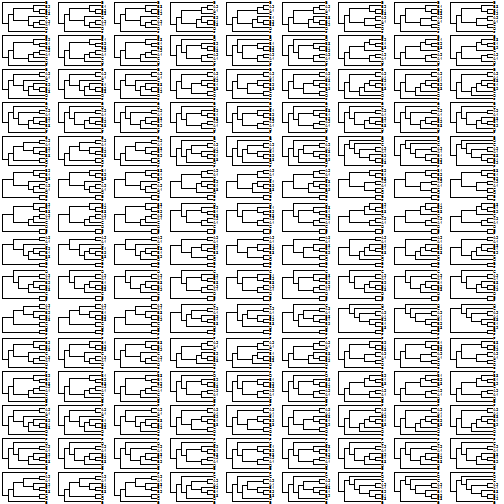## Tuesday, August 9, 2016

### More on resolving multifurcating nodes in all possible ways

I just pushed an update to phytools on GitHub that contains the functions to resolve one or all multifurcating nodes in all possible ways that I described here late last night.

Note that, as stated in the function documentation:

For `resolveNode` applied to a multifurcation with n descendants, the number of resolved trees will be equal to the number of possible rooted trees of n taxa. (For instance, three for a trifurcation, 15 for a quadrifurcation, and so on.)

For `resolveAllNodes` the number of fully resolved trees will be equal to the product of numbers for `resolveNode` applied to each multifurcation separately. (For instance, 45 for a tree containing one trifurcation and one quadrifurcation.)

Let's try it to see what I mean.

Here is a tree with three multifurcations - two are trifurcations, and the third is a quadrifurcation:

``````library(phytools) ## GitHub version 0.5.45
``````The number of possible fully resolved trees should thus be:

``````3*3*15
``````
``````##  135
``````

Let's try:

``````trees<-resolveAllNodes(tree)
trees
``````
``````## 135 phylogenetic trees
``````
``````par(mfrow=c(15,9))
plotTree(trees,lwd=1,fsize=0.5)
``````Cool. Note that the number of possible trees increases rapidly with the number of multifurcations and, especially, with the degree of the multifurcations. For instance, adding one quadrifurcation is much worse than adding two trifurcations, and so on.

That's it.